# Voronoi art

Voronoi diagrams are commonly thought of in terms of a set of points. Given a set of points {Pi}, you cover the plane with disjoint convex tiles {Ti} such that Tk contains all the points in the plane which are closer to pk than any other pi. This has many practical applications, and there are well-known algorithms for finding the Voronoi diagram of a set of points.

My interest is in applying and extending this concept to produce attractive ornamental designs. It turns out that taking the Voronoi diagram of an interesting set of points tends to yield an interesting tiling of the plane! I've written a program that renders Voronoi diagrams of sets of points to show you what I mean. For each pair of illustrations below, the diagram on the left is a schematic showing the set of points used to generate the rendered tiling on the right. Note that in each case, the tilings were produced using only overlapping square lattices.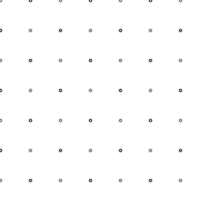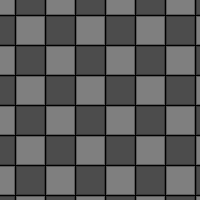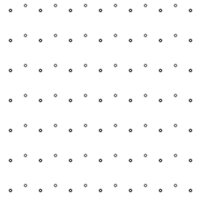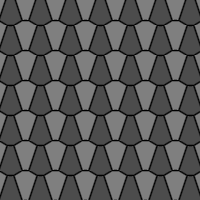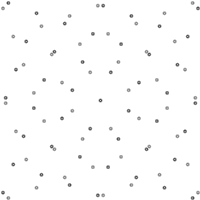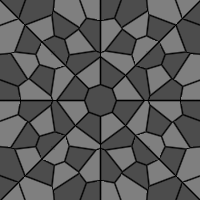But this concept can be made more general. In particular, the pi don't have to be points. They can be arbitrary subsets of the plane. The mathematical construction is the same: Tk still contains all the points closer to pk than any other pi. You just need to be more intelligent about computing distances from objects. As a canonical example, consider the Voronoi diagram induced by a point and a line: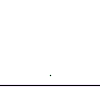This will induce two disjoint regions in the plane. If you remember the construction of conic sections, a parabola can be defined as the locus of points equidistant from a focus and a line. Sure enough, we get the following Voronoi diagram: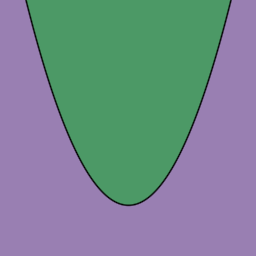I've extended the basic renderer mentioned above to implement this more general Voronoi diagram drawing concept. It can create some beautiful, varied and often unexpected pictures. Here's my favorite: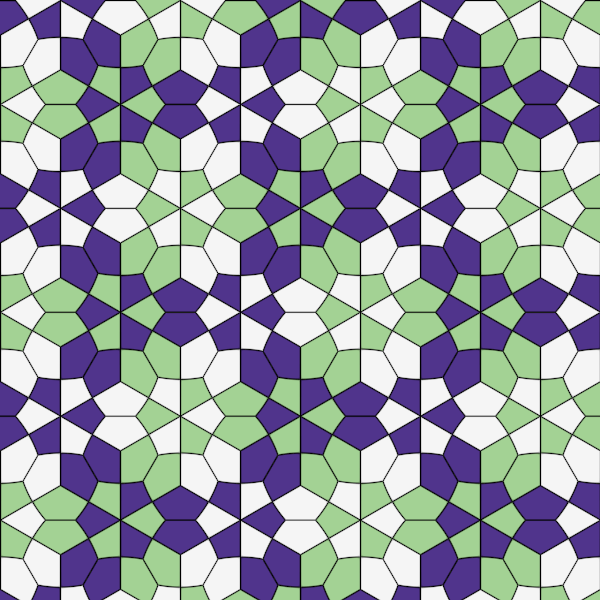It was created by taking the Voronoi diagram of coloured rings, interlocked in a hexagonal pattern. Here's a schematic, showing the elements {pi} that induced the above picture.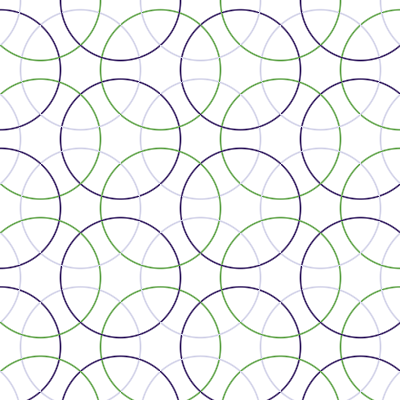## Gallery

All images are copyright 2000 by Craig S. Kaplan. You are free to use them for personal and non-commercial purposes. Please check with me about any other uses.

## Other resources

• Jos Leys has adapted the ideas presented here, creating a Voronoi diagram renderer in Ultrafractal. I invite you to visit his gallery for many more examples like the ones given here.